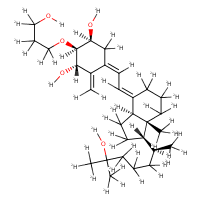Structure InformationCompound Identification

SMILES

[2H]OC([2H])([2H])C([2H])([2H])C([2H])([2H])O[C@@]1([2H])[C@]([2H])(O[2H])C(=C([2H])[2H])\C(=C(\[2H])/C(/[2H])=C2\C([2H])([2H])C([2H])([2H])C([2H])([2H])[C@@]3(C([2H])([2H])[2H])[C@@]2([2H])C([2H])([2H])C([2H])([2H])[C@]3([2H])[C@@]([2H])(C([2H])([2H])[2H])C([2H])([2H])C([2H])([2H])C([2H])([2H])C(O[2H])(C([2H])([2H])[2H])C([2H])([2H])[2H])C([2H])([2H])[C@@]1([2H])O[2H]

InChIKey

InChIKey=FZEXGDDBXLBRTD-JPWHAGTBSA-N

Formula

C30H50O5

Mass

541.03

### Export to:

Taxonomic Classification

Taxonomy Tree

Kingdom

Organic compounds

Superclass

Lipids and lipid-like molecules

Class

Steroids and steroid derivatives

Subclass

Vitamin D and derivatives

Intermediate Tree Nodes

Not available

Direct Parent

Vitamin D and derivatives

Alternative Parents

Molecular Framework

Aliphatic homopolycyclic compounds

Substituents

Triterpenoid - Cyclitol or derivatives - Tertiary alcohol - Cyclic alcohol - Secondary alcohol - Ether - Dialkyl ether - Organic oxygen compound - Hydrocarbon derivative - Primary alcohol - Organooxygen compound - Alcohol - Aliphatic homopolycyclic compound

Description

This compound belongs to the class of organic compounds known as vitamin d and derivatives. These are compounds containing a secosteroid backbone, usually secoergostane or secocholestane.

External Descriptors

Not available